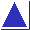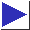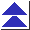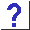# comp.lang.c FAQ list ·Question 6.16

Q: How can I dynamically allocate a multidimensional array?

A: The traditional solution is to allocate an array [footnote] of pointers to pointers, and then initialize each pointer to a dynamically-allocated ``row.'' Here is a two-dimensional example:

```	#include <stdlib.h>

int **array1 = malloc(nrows * sizeof(int *));
for(i = 0; i < nrows; i++)
array1[i] = malloc(ncolumns * sizeof(int));
```
(In real code, of course, all of malloc's return values would be checked. You can also use sizeof(*array1) and sizeof(**array1) instead of sizeof(int *) and sizeof(int); see [footnote] .)

You can keep the array's contents contiguous, at the cost of making later reallocation of individual rows more difficult, with a bit of explicit pointer arithmetic:

```	int **array2 = malloc(nrows * sizeof(int *));
array2 = malloc(nrows * ncolumns * sizeof(int));
for(i = 1; i < nrows; i++)
array2[i] = array2 + i * ncolumns;
```
In either case (i.e for array1 or array2), the elements of the dynamic array can be accessed with normal-looking array subscripts: arrayx[i][j] (for 0 <= i < nrows and 0 <= j < ncolumns). Here is a schematic illustration of the layout of array1 and array2: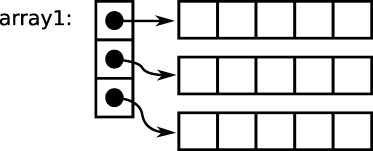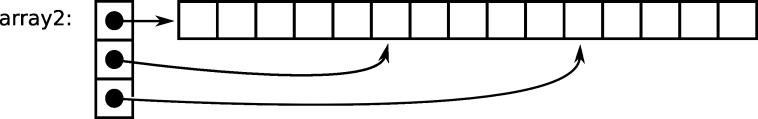If the double indirection implied by the above schemes is for some reason unacceptable,[footnote] you can simulate a two-dimensional array with a single, dynamically-allocated one-dimensional array:

```	int *array3 = malloc(nrows * ncolumns * sizeof(int));
```
However, you must now perform subscript calculations manually, accessing the i,jth element with the expression
```array3[i * ncolumns + j]
```
and this array cannot necessarily be passed to functions which expect multidimensional arrays. (A macro such as
```	#define Arrayaccess(a, i, j) ((a)[(i) * ncolumns + (j)])
```
could hide the explicit calculation, but invoking it would require parentheses and commas which wouldn't look exactly like conventional C multidimensional array syntax, and the macro would need access to at least one of the dimensions, as well. See also question 6.19.)

Yet another option is to use pointers to arrays:

```	int (*array4)[NCOLUMNS] = malloc(nrows * sizeof(*array4));
```
or even
```	int (*array5)[NROWS][NCOLUMNS] = malloc(sizeof(*array5));
```
but the syntax starts getting horrific (accesses to array5 look like (*array5)[i][j]), and at most one dimension may be specified at run time.

With all of these techniques, you may of course need to remember to free the arrays when they are no longer needed; in the case of array1 and array2 this takes several steps (see also question 7.23):

```	for(i = 0; i < nrows; i++)
free((void *)array1[i]);
free((void *)array1);

free((void *)array2);
free((void *)array2);
```
Also, you cannot necessarily intermix dynamically-allocated arrays with conventional, statically-allocated ones (see question 6.20, and also question 6.18).

Finally, in C99 you can use a variable-length array.

All of these techniques can also be extended to three or more dimensions. Here is a three-dimensional version of the first technique (which, like the rest of the fragments presented here, requires error-checking before being used in a real program):

```	int ***a3d = (int ***)malloc(xdim * sizeof(int **));
for(i = 0; i < xdim; i++) {
a3d[i] = (int **)malloc(ydim * sizeof(int *));
for(j = 0; j < ydim; j++)
a3d[i][j] = (int *)malloc(zdim * sizeof(int));
}
```